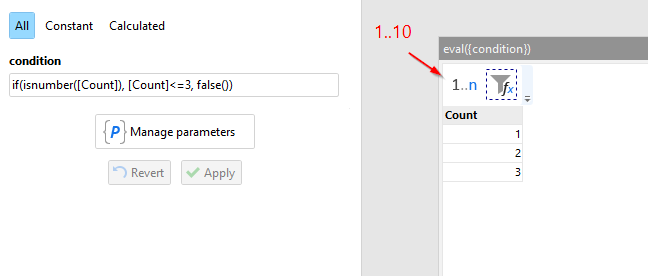# Eval() does not work

Hi

When I use the following expression in a conditional filter, it works. However when I put the expression in a text parameter it does not work. What is the problem here?//This works

``````eval(
'
if(
istext([AARD_WERKEN]), //check data type om problemen met empty() values te vermijden
if(
in([AARD_WERKEN], "A;B;C;X", ";"), //selecteren mogelijke aard van de werken
true(),
false()
),
false()
)
')
``````

//This does not work.
//So here the parameter has the value of the expression above (without the eval() of course).

``````eval(
{filter_aard_werken}
)
``````

It throws the error:

``````Error: Condition must evaluate to either TRUE or FALSE. However in row #1 it's: if(
istext([AARD_WERKEN]), //check data type om problemen met empty() values te vermijden
if(
in([AARD_WERKEN], "A;B;C;X", ";"), //selecteren mogelijke aard van de werken
true(),
false()
),
false()
).
Source: action "Filter by condition", module "bereken_sheet", table "filteren"

``````

Hmm… It works for me.Make sure the contents of the parameter is correct - no typos, extra characters, etc.

Also, you can simplify

``````if(
in([AARD_WERKEN], "A;B;C;X", ";"), //selecteren mogelijke aard van de werken
true(),
false()
)
``````

down to (no if() required)

``````in([AARD_WERKEN], "A;B;C;X", ";"), //selecteren mogelijke aard van de werken
``````

because in() by itself returns either TRUE or FALSE. Your expression basically tells IF TRUE THEN TRUE, IF FALSE THEN FALSE.

Hi

I have the impession that when I put the expression in a text parameter the indentation in the expression causes the issue.
When I put it on a single line keeping the indentation / comments, it does not work. When I put it on a sinle line removing indentation, it seems to work.

//This does not work

``````if(     isnumber([E_PEIL]), //check data type     if(         [E_PEIL] < 41, //Check de conditie als data type number is         true(),         false()     ),     false() )
``````

//This works

``````if(isnumber([E_PEIL]),if([E_PEIL] < 41,true(),false()),false())
``````

Additional question: how can I put multiple lines in a text param? I cannot enter a new line manually but during iteration, I can see that easymorph accepts multiline parameter values?

A comment ends where the line ends. In other words, everything after `//` is ignored. Remove the comments to make it work.

Not possible with the standard input fields. Although, you can concatenate a multiline value from a few rows and then pass it to a module parameter with the “Call” action.

yes indeed thanks!